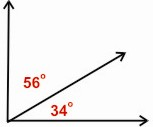HOME MATH DICTIONARY DOWNLOAD FEEDBACK DISCLAIMER
 Question: What are Complementary Angles ? Answer: Two Angles are Complementary if they add up to 90 degrees.In a triangle with a right angle the other two angles are complementary: angle A + angle C = 90°. If the two complementary angles are adjacent (i.e. have a common vertex and share a side, but do not have any interior points in common) their non-shared sides form a right angle. In Euclidean geometry, the two acute angles in a right triangle are complementary, because there are 180° in a triangle and 90° have been accounted for by the right angle.